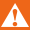Trade Responsibly. Trade Responsibly.CFDs are complex instruments and come with a high risk of losing money rapidly due to leverage. 78.90% of retail investor accounts lose money when trading CFDs with this provider. You should consider whether you understand how CFDs work and whether you can afford to take the high risk of losing your money.CFDs and Spread Betting are complex instruments and come with a high risk of losing money rapidly due to leverage. 80.59% of retail investor accounts lose money when trading CFDs and Spread Betting with this provider. You should consider whether you understand how CFDs and Spread Betting work and whether you can afford to take the high risk of losing your money.
Invest Responsibly: Trading CFDs involves significant risks.# Forex Swap

A key piece of trading terminology that you will come across and may struggle to understand if you are new to trading, is Swap (also called rollover). It is important to be aware of what it means and how it works, or you may get quite the shock the first time you leave a trade open overnight! In this article, we will explain the concept of swaps, what it means and how it is applied to your positions.

## What is forex swap?

So, how does an fx swap work? Well as you probably already know when you trade currencies, you are purchasing one underlying currency and simultaneously selling the other, and because each currency has a unique interest rate, there will naturally be a difference between the two and this is how the forex swap is derived.

Swap/rollover is an automatic charge (or a payment) incurred when you leave a trade open overnight and can be positive or negative depending on the interest rate differential of the pair, and the direction of the trade.

Each pair has a Long rate (Buy position) and a Short rate (Sell position) and these are updated by FxPro accordingly.

## How to calculate swap in forex?

When determining swap in forex, the cost or income ‘rate’ calculation is based on the interest rate differential between the Tomorrow Next Deposit Rates(TNDR) of the 2 currencies in question, plus an additional rate charged by the Company.

Swap/Rollover is calculated once per weekday that the position is left open overnight, and a triple charge on Friday to account for positions left open during the weekend.

To calculate the applied swap in forex CFD positions we use the following calculation:

Forex Swap = (Pip Value * Swap Rate * Number of Nights) / 10

*In FX we divide by 10 due to the fact that our fx swap rates are stated in points (not pips)

We can demonstrate this with an example:

Pair: EURUSD

Direction: LONG

Number of nights left open: 3

Let's assume the current swap rates for EURUSD are as follows:

Short= -0.8052 Points  / Long= -4.8313 Points

A €100,000 unit trade means we have a pip value of \$10. So according to the formula above, we can calculate the following:

(10 * -4.8313 * 3) / 10 = -14.49 USD Swap charge

Swap fees are converted into the base currency of your trading account. With FxPro, rollover takes place at 10 pm UK time, which means that to avoid an fx swap you should close your position prior to this.

You can check the current swap rates for each instrument with FxPro in the website or platform specifications and we also have a useful swap calculator.

## How to calculate non fx swap?

As well as fx swap, swap also applies to other tradeable assets, for example, CFDs on Metals, Indices, Energies & Shares. (FxPro does not apply swap on Future contracts).

Other instruments have a slightly different calculation in swap:

Lot Size * Swap Rate * Number of Nights

For example:

Instrument: GOLD

Direction: SHORT

Number of nights left open: 2

Let's assume the current swap rates for GOLD are as follows:

Short= -1.24 Points / Long=  -3.79 Points

The calculation for this position would be:

1 * -1.24 * 2 = -2.48 USD swap charge.

The result will be in the currency that the instrument is denominated, in this case, USD.

## What is the difference between fx and currency swap?

Although either of the terms can generally be used to describe the process of fx swaps in CFD trading, there are actually two different types in swap: fx swaps and currency swaps.

For the sake of clarity, let us briefly explain what each means.

What we have explained above and what applies to FxPro CFDs is foreign exchange swaps, which as we know, is an agreement between two parties to exchange an amount of one currency for an equal amount of another.

Currency swaps, on the other hand, apply more to long term investors and hedge funds. A currency swap involves two concurrent currency purchases. One of the investments happens through a forward contract while the other occurs at the spot rate. Various market participants, including financial institution and institutional investors, utilise currency swaps for hedging foreign exchange positions or to reduce borrowing costs.

We hope that you now have a better idea of what swap means in CFD trading.